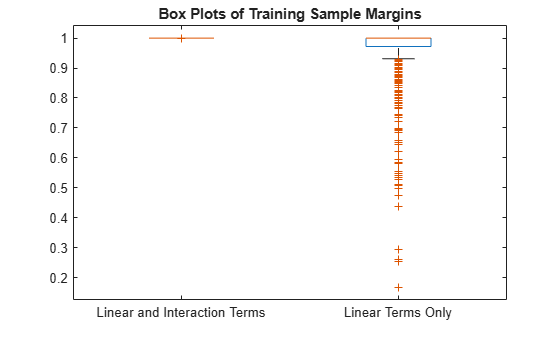# resubEdge

Resubstitution classification edge

## Syntax

``e = resubEdge(Mdl)``
``e = resubEdge(Mdl,'IncludeInteractions',includeInteractions)``

## Description

example

````e = resubEdge(Mdl)` returns the weighted resubstitution Classification Edge (`e`) for the trained classification model `Mdl` using the predictor data stored in `Mdl.X`, the corresponding true class labels stored in `Mdl.Y`, and the observation weights stored in `Mdl.W`.```

example

````e = resubEdge(Mdl,'IncludeInteractions',includeInteractions)` specifies whether to include interaction terms in computations. This syntax applies only to generalized additive models.```

## Examples

collapse all

Load the `ionosphere` data set. This data set has 34 predictors and 351 binary responses for radar returns, either bad (`'b'`) or good (`'g'`).

`load ionosphere`

Train a support vector machine (SVM) classifier. Standardize the data and specify that `'g'` is the positive class.

`SVMModel = fitcsvm(X,Y,'Standardize',true,'ClassNames',{'b','g'});`

`SVMModel` is a trained `ClassificationSVM` classifier.

Estimate the resubstitution edge, which is the mean of the training sample margins.

`e = resubEdge(SVMModel)`
```e = 5.0997 ```

The classifier edge measures the average of the classifier margins. One way to perform feature selection is to compare training sample edges from multiple models. Based solely on this criterion, the classifier with the highest edge is the best classifier.

Load the `ionosphere` data set. Remove the first two predictors for stability.

```load ionosphere X = X(:,3:end);```

Define these two data sets:

• `fullX` contains all predictors.

• `partX` contains the 10 most important predictors.

```fullX = X; idx = fscmrmr(X,Y); partX = X(:,idx(1:10));```

Train a naive Bayes classifier for each predictor set.

```FullMdl = fitcnb(fullX,Y); PartMdl = fitcnb(partX,Y);```

`FullMdl` and `PartMdl` are trained `ClassificationNaiveBayes` classifiers.

Estimate the training sample edge for each classifier.

`fullEdge = resubEdge(FullMdl)`
```fullEdge = 0.6554 ```
`partEdge = resubEdge(PartMdl)`
```partEdge = 0.7796 ```

The edge of the classifier trained on the 10 most important predictors is larger. This result suggests that the classifier trained using only those predictors has a better in-sample fit.

Compare a generalized additive model (GAM) with linear terms to a GAM with both linear and interaction terms by examining the training sample margins and edge. Based solely on this comparison, the classifier with the highest margins and edge is the best model.

Load the 1994 census data stored in `census1994.mat`. The data set consists of demographic data from the US Census Bureau to predict whether an individual makes over \$50,000 per year. The classification task is to fit a model that predicts the salary category of people given their age, working class, education level, marital status, race, and so on.

`load census1994`

`census1994` contains the training data set `adultdata` and the test data set `adulttest`. To reduce the running time for this example, subsample 500 training observations from `adultdata` by using the `datasample` function.

```rng('default') % For reproducibility NumSamples = 5e2; adultdata = datasample(adultdata,NumSamples,'Replace',false);```

Train a GAM that contains both linear and interaction terms for predictors. Specify to include all available interaction terms whose p-values are not greater than 0.05.

`Mdl = fitcgam(adultdata,'salary','Interactions','all','MaxPValue',0.05)`
```Mdl = ClassificationGAM PredictorNames: {1x14 cell} ResponseName: 'salary' CategoricalPredictors: [2 4 6 7 8 9 10 14] ClassNames: [<=50K >50K] ScoreTransform: 'logit' Intercept: -28.5594 Interactions: [82x2 double] NumObservations: 500 Properties, Methods ```

`Mdl` is a `ClassificationGAM` model object. `Mdl` includes 82 interaction terms.

Estimate the training sample margins and edge for `Mdl`.

```M = resubMargin(Mdl); E = resubEdge(Mdl)```
```E = 1.0000 ```

Estimate the training sample margins and edge for `Mdl` without including interaction terms.

```M_nointeractions = resubMargin(Mdl,'IncludeInteractions',false); E_nointeractions = resubEdge(Mdl,'IncludeInteractions',false)```
```E_nointeractions = 0.9516 ```

Display the distributions of the margins using box plots.

```boxplot([M M_nointeractions],'Labels',{'Linear and Interaction Terms','Linear Terms Only'}) title('Box Plots of Training Sample Margins')```When you include the interaction terms in the computation, all the resubstitution margin values for `Mdl` are 1, and the resubstitution edge value (average of the margins) is 1. The margins and edge decrease when you do not include the interaction terms in `Mdl`.

## Input Arguments

collapse all

Classification machine learning model, specified as a full classification model object, as given in the following table of supported models.

ModelClassification Model Object
Generalized additive model`ClassificationGAM`
k-nearest neighbor model`ClassificationKNN`
Naive Bayes model`ClassificationNaiveBayes`
Neural network model`ClassificationNeuralNetwork`
Support vector machine for one-class and binary classification`ClassificationSVM`

Flag to include interaction terms of the model, specified as `true` or `false`. This argument is valid only for a generalized additive model (GAM). That is, you can specify this argument only when `Mdl` is `ClassificationGAM`.

The default value is `true` if `Mdl` contains interaction terms. The value must be `false` if the model does not contain interaction terms.

Data Types: `logical`

collapse all

### Classification Edge

The classification edge is the weighted mean of the classification margins.

One way to choose among multiple classifiers, for example to perform feature selection, is to choose the classifier that yields the greatest edge.

### Classification Margin

The classification margin for binary classification is, for each observation, the difference between the classification score for the true class and the classification score for the false class. The classification margin for multiclass classification is the difference between the classification score for the true class and the maximal classification score for the false classes.

If the margins are on the same scale (that is, the score values are based on the same score transformation), then they serve as a classification confidence measure. Among multiple classifiers, those that yield greater margins are better.

## Algorithms

`resubEdge` computes the classification edge according to the corresponding `edge` function of the object (`Mdl`). For a model-specific description, see the `edge` function reference pages in the following table.

ModelClassification Model Object (`Mdl`)`edge` Object Function
Generalized additive model`ClassificationGAM``edge`
k-nearest neighbor model`ClassificationKNN``edge`
Naive Bayes model`ClassificationNaiveBayes``edge`
Neural network model`ClassificationNeuralNetwork``edge`
Support vector machine for one-class and binary classification`ClassificationSVM``edge`

## Version History

Introduced in R2012a

expand all